# Solution assignment 06 Logarithmic functions and graphs

### Assignment 6

Using their graphs show that the following functions (a logarithmic and an exponential function):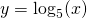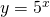are inverse functions. Which line is the symmetry axis?

### Solution

In the figure both functions are sketched based on a number of points. For the logarithmic function these points we take: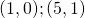and for the exponential function: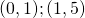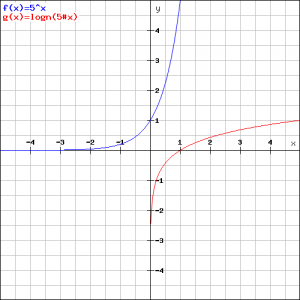We notice that the graph of one function can be obtained by the other by exchanging the variablesand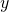. Therefore the symmetry axis is the line: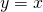. We can see this in the figure above.

0